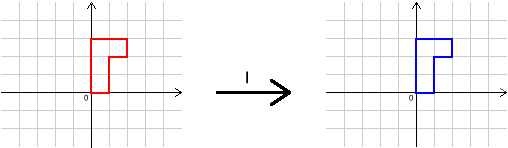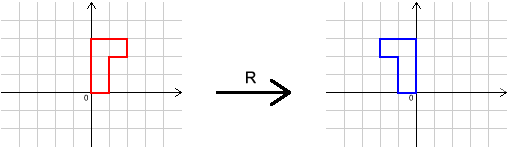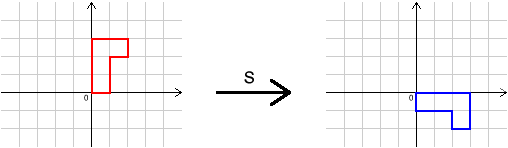#### You may also like### Chess

What would be the smallest number of moves needed to move a Knight from a chess set from one corner to the opposite corner of a 99 by 99 square board?### 2001 Spatial Oddity

With one cut a piece of card 16 cm by 9 cm can be made into two pieces which can be rearranged to form a square 12 cm by 12 cm. Explain how this can be done.### Screwed-up

A cylindrical helix is just a spiral on a cylinder, like an ordinary spring or the thread on a bolt. If I turn a left-handed helix over (top to bottom) does it become a right handed helix?

# Simplifying Transformations

##### Age 11 to 14 Challenge Level:

This problem follows on from Combining Transformations .

We shall concentrate on the transformations $I$, $R$ and $S$ in this question. Here they are again:We can combine $R$, $S$, $R^{-1}$ and $S^{-1}$ in lots of different ways.
$S S R S R^{-1} S R S R^{-1}$ and $S^{-1} R R S R S R R^{-1} S R^{-1}$ are two examples of transformations obtained like this.

In fact, there are infinitely many ways to combine them.

How many different transformations can you find made up from combinations of $R$, $S$ and their inverses?
(We shall count two transformations as the same if they have the same effect on all starting shapes.)
Can you be sure that you have found them all?

Convince yourself that $R S = S^3 R$.

In Combining Transformations you found simpler expressions for powers of $R$ and $S$.
Use these and $R S = S^3 R$ to simplify $S S R S R^{-1} S R S R^{-1}$ and $S^{-1}R R S R S R R^{-1} S R^{-1}$.

You might like to look at the article Grouping Transformations , which explains some of the mathematics behind these problems.